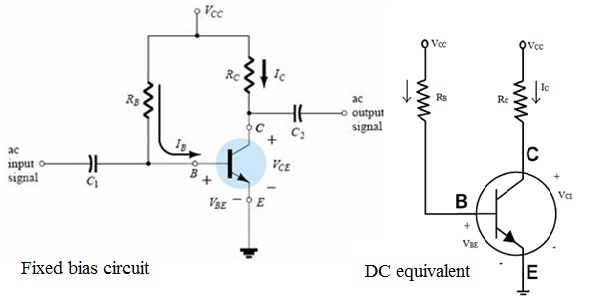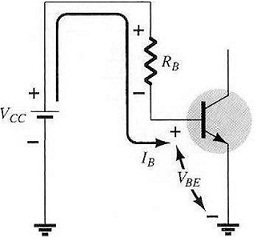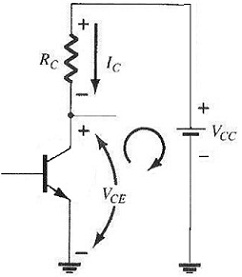#### Bias Stability, Physics tutorial

Fixed Bias Circuit:

The fixed-bias circuit gives relatively straightforward and simple introduction to transistor dc bias analysis. Although network uses npn transistor, equations and calculations apply evenly well to pnp transistor configuration just by changing all current direction and voltage polarities. Current direction of is really current directions, and voltages are stated by standard double-subscript notation. For dc analysis network can be isolated from indicated ac level by replacing capacitor with the open circuit equivalent. Additionally, dc supply Vcc can be separated in two supplies (for analysis purpose only) as shown in figure to allow a separation of input and output circuits. It also decreases linkage between two to base current IB. Separation is surely valid; VCC is connected directly to RB and RC.Forward Bias Base Emitter:
Consider first base-emitter circuit loop. Writing Kirchhoff's voltage equation in clockwise direction for loop, we get

+VCC - IBRB - VBE = 0Note polarity of voltage drop across RB as established by indicated direction of IB. Solving equation for current IB will result in following

IB= (VCC- VBE)/RB

As supply voltage VCC and base-emitter voltage VBE are constant, selection of base resistor, RB, sets level of base current for operating point.

Collector-Emitter Loop:Collector-emitter section of network with indicated direction of current IC and resulting polarity across RC. Magnitude of collector current is related directly to IB through

IC = βIB

It is interesting to note that as base current is controlled by level of RB and IC is related to IB by constant β, magnitude of IC is not function of resistance RC. Change RC to any level and it will not affect level of IB or IC as long as we remain in active region of device. Though, level of RC will determine magnitude of VCE that is significant parameter. Applying Kirchhoff's voltage law in clockwise direction around indicated closed loop will result in following:

VCE + ICRC - VCC = 0 and VCE = VCC - ICRC

It defines that voltage across collector-emitter region of the transistor in fixed-bias configuration is supply voltage less the drop across RC.

VCE = VC - VE

Where VCE is voltage from collector to emitter and VC and VE are voltages from collector and emitter to ground respectively. But in this situation, as VE = 0V, we have

VCE= VC

In addition, as VBE= VB- VE and VE = 0V, then VBE= VB

Emitter-Stabilized Bias Circuit:

The dc bias network contains emitter resistor to enhance stability level over that of fixed-bias configuration. Analysis will be performed by first examining base-emitter loop and then using result to investigate collector-emitter loop.

Base-emitter loop of network can be redrawn as shown. Writing Kirchhoff's voltage law around indicated loop in clockwise direction will result in given equations:

+VCC - IBRB - VBE - IERE = 0

Recall that IE=(β +1)IB

Substituting equation will result in

VCC- IBRB- VBE-(β+1)IBRE=0

Expanding and multiplying through by (-1) we have

IBRB+β+1RE-VCC+ VBE=0

And solving for gives

IB= VCC- VBERB+(β+1)RE

Collector-Emitter Loop:

Writing Kirchhoff's voltage law for indicated loop in clockwise direction will result in

+IERE + VCE + ICRC - VCC = 0

Substituting IE ≈ IC and regrouping terms provides

VCE - VCC + IC(RC  +RE) = 0 and

VCE = VCC - IC(RC + RE)

Single subscript voltage VE is voltage from emitter to ground and is determined by

VE = IERE

While voltage from collector to ground can be determined from

VCE = VC - VE and VC = VCE + VE or VC = VCC - ICRC

The voltage at the base with respect to ground can be determined from

VB = VCC - IBRB

VB = VBE + VE

Voltage Divider Bias:

The bias current ICQ and voltage VCEQ were function of current Gain (β) of te transistor. Though, since β is temperature sensitive, especially for silicon transistors, and the actual value of beta is usually not well defined, it will be desirable to develop a bias circuit that is less dependent, or in fact, independent of the transistor beta. If analyzed on the exact basis sensitivity to changes in beta is quite small. If circuit parameters are properly chosen, resulting level of ICQ and VCEQ can be almost totally independent of beta. A Q-point is stated by fixed level of ICQ and VCEQ. The level IEQ will change with change in beta, but operating point on characteristic defined by ICQ and VCEQ can remain fixed if proper circuit parameters are used above, there are two methods which can be applied to examine voltage-divider configuration.

Exact Analysis:

The Thevenin equivalent network for network to left of base terminal can be found in given manner:

RTh: Voltage source is replaced by the short-circuit equivalent.

RTh = R1||R2

ETh: Voltage source VCC is returned to network and open- circuit Thevenin voltage determined as follows: Applying voltage-divider rule:

ETh = VR2 = R2VCC/(R1 + R2)

Thevenin network is redrawn, and IBQ can be determined by first applying Kirchhoff's voltage law in clockwise direction for loop indicated:

ETh - IBRTh - VBE - IERE = 0

Substituting IE = (β + 1)IB and solving for IB yields

IB = (ETh - VBE)/(RTh + (β + 1)RE)

The numerator is again a difference of two voltage levels and denominator is base resistance plus the emitter resistor reflected by (β + 1) certainly very similar to equation in base-emitter loop previously treated.

Once IB is known, remaining quantities of network can be found in same manner as developed for emitter-bias configuration. That is,

VCE = VCC - IC(RC + RE)

Approximate Analysis:

The input section of voltage-divider configuration can be represented by network of resistance Ri is equivalent resistance between base and ground for transistor with the emitter resistor RE. Recall that Ri = (β + 1)RE. If Ri is much larger than resistance R2, current IB will be much smaller than I2 and I2 will be approximately equal to I1.

Voltage across R2 that is actually base voltage can be determined using voltage-divider rule (therefore name for configuration). That is:

VE = R2VCC/(R1 + R2)

Since R1 = (β + 1)RE ≈ βRE the condition which will define whether approximate approach can be applied will be following:

βRE ≥ 10R2

In other words, if β times the value of RE is at least 10 times value of R2, the approximate approach can be applied with high degree of accuracy.

Once VB is determined, level of VE can be calculated from

VE = VB - VBE

And emitter current can be determined from:

IE = VE/RE

And

ICQ ≈ IE

Collector-to-emitter voltage is determined by

VCE = VCC - ICRC - IERE

But since IE ≈ IC

VCEQ = VCC - IC(RC + RE)

Tutorsglobe: A way to secure high grade in your curriculum (Online Tutoring)